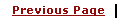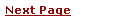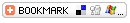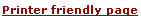# - Unix, Linux Command# NAME

rsautl - RSA utility

# SYNOPSIS

openssl rsautl [-in file] [-out file] [-inkey file] [-pubin] [-certin] [-sign] [-verify] [-encrypt] [-decrypt] [-pkcs] [-ssl] [-raw] [-hexdump] [-asn1parse]

# DESCRIPTION

The rsautl command can be used to sign, verify, encrypt and decrypt data using the RSA algorithm.

# COMMAND OPTIONS

TagDescription
-in filename This specifies the input filename to read data from or standard input if this option is not specified.
-out filename specifies the output filename to write to or standard output by default.
-inkey file the input key file, by default it should be an RSA private key.
-pubin the input file is an RSA public key.
-certin the input is a certificate containing an RSA public key.
-sign sign the input data and output the signed result. This requires and RSA private key.
-verify verify the input data and output the recovered data.
-encrypt encrypt the input data using an RSA public key.
-decrypt decrypt the input data using an RSA private key.
-pkcs, -oaep, -ssl, -raw the padding to use: PKCS#1 v1.5 (the default), PKCS#1 OAEP, special padding used in SSL v2 backwards compatible handshakes, or no padding, respectively. For signatures, only -pkcs and -raw can be used.
-hexdump hex dump the output data.
-asn1parse asn1parse the output data, this is useful when combined with the -verify option.

# NOTES

rsautl because it uses the RSA algorithm directly can only be used to sign or verify small pieces of data.

# EXAMPLES

Sign some data using a private key:

 ``` openssl rsautl -sign -in file -inkey key.pem -out sig ```

Recover the signed data

 ``` openssl rsautl -verify -in sig -inkey key.pem ```

Examine the raw signed data:

 ``` openssl rsautl -verify -in file -inkey key.pem -raw -hexdump ```

 ``` 0000 - 00 01 ff ff ff ff ff ff-ff ff ff ff ff ff ff ff ................ 0010 - ff ff ff ff ff ff ff ff-ff ff ff ff ff ff ff ff ................ 0020 - ff ff ff ff ff ff ff ff-ff ff ff ff ff ff ff ff ................ 0030 - ff ff ff ff ff ff ff ff-ff ff ff ff ff ff ff ff ................ 0040 - ff ff ff ff ff ff ff ff-ff ff ff ff ff ff ff ff ................ 0050 - ff ff ff ff ff ff ff ff-ff ff ff ff ff ff ff ff ................ 0060 - ff ff ff ff ff ff ff ff-ff ff ff ff ff ff ff ff ................ 0070 - ff ff ff ff 00 68 65 6c-6c 6f 20 77 6f 72 6c 64 .....hello world ```

The PKCS#1 block formatting is evident from this. If this was done using encrypt and decrypt the block would have been of type 2 (the second byte) and random padding data visible instead of the 0xff bytes.

It is possible to analyse the signature of certificates using this utility in conjunction with asn1parse. Consider the self signed example in certs/pca-cert.pem . Running asn1parse as follows yields:

 ``` openssl asn1parse -in pca-cert.pem ```

 ``` 0:d=0 hl=4 l= 742 cons: SEQUENCE 4:d=1 hl=4 l= 591 cons: SEQUENCE 8:d=2 hl=2 l= 3 cons: cont [ 0 ] 10:d=3 hl=2 l= 1 prim: INTEGER :02 13:d=2 hl=2 l= 1 prim: INTEGER :00 16:d=2 hl=2 l= 13 cons: SEQUENCE 18:d=3 hl=2 l= 9 prim: OBJECT :md5WithRSAEncryption 29:d=3 hl=2 l= 0 prim: NULL 31:d=2 hl=2 l= 92 cons: SEQUENCE 33:d=3 hl=2 l= 11 cons: SET 35:d=4 hl=2 l= 9 cons: SEQUENCE 37:d=5 hl=2 l= 3 prim: OBJECT :countryName 42:d=5 hl=2 l= 2 prim: PRINTABLESTRING :AU .... 599:d=1 hl=2 l= 13 cons: SEQUENCE 601:d=2 hl=2 l= 9 prim: OBJECT :md5WithRSAEncryption 612:d=2 hl=2 l= 0 prim: NULL 614:d=1 hl=3 l= 129 prim: BIT STRING ```

The final BIT STRING contains the actual signature. It can be extracted with:

 ``` openssl asn1parse -in pca-cert.pem -out sig -noout -strparse 614 ```

The certificate public key can be extracted with:

 ``` openssl x509 -in test/testx509.pem -pubkey -noout >pubkey.pem ```

The signature can be analysed with:

 ``` openssl rsautl -in sig -verify -asn1parse -inkey pubkey.pem -pubin ```

 ``` 0:d=0 hl=2 l= 32 cons: SEQUENCE 2:d=1 hl=2 l= 12 cons: SEQUENCE 4:d=2 hl=2 l= 8 prim: OBJECT :md5 14:d=2 hl=2 l= 0 prim: NULL 16:d=1 hl=2 l= 16 prim: OCTET STRING 0000 - f3 46 9e aa 1a 4a 73 c9-37 ea 93 00 48 25 08 b5 .F...Js.7...H%.. ```

This is the parsed version of an ASN1 DigestInfo structure. It can be seen that the digest used was md5. The actual part of the certificate that was signed can be extracted with:

 ``` openssl asn1parse -in pca-cert.pem -out tbs -noout -strparse 4 ```

and its digest computed with:

 ``` openssl md5 -c tbs MD5(tbs)= f3:46:9e:aa:1a:4a:73:c9:37:ea:93:00:48:25:08:b5 ```

which it can be seen agrees with the recovered value above.